##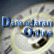Dividend Regressions: January 2022

Variables used in the regression

1. Dividend Yield = Dividends per share in most recent year/ Current Stock Price
2. Dividend Payout Ratio = Dividends / Net Income
3. Beta: Regression or Bottom up beta
4. Expected Growth in EPS over next 5 years = Consensus analyst estimate (or your own) of expected growth in EPS . If you don't have an analyst estimate, use your own estimate of expected growth.

US Regression: Dividend Yield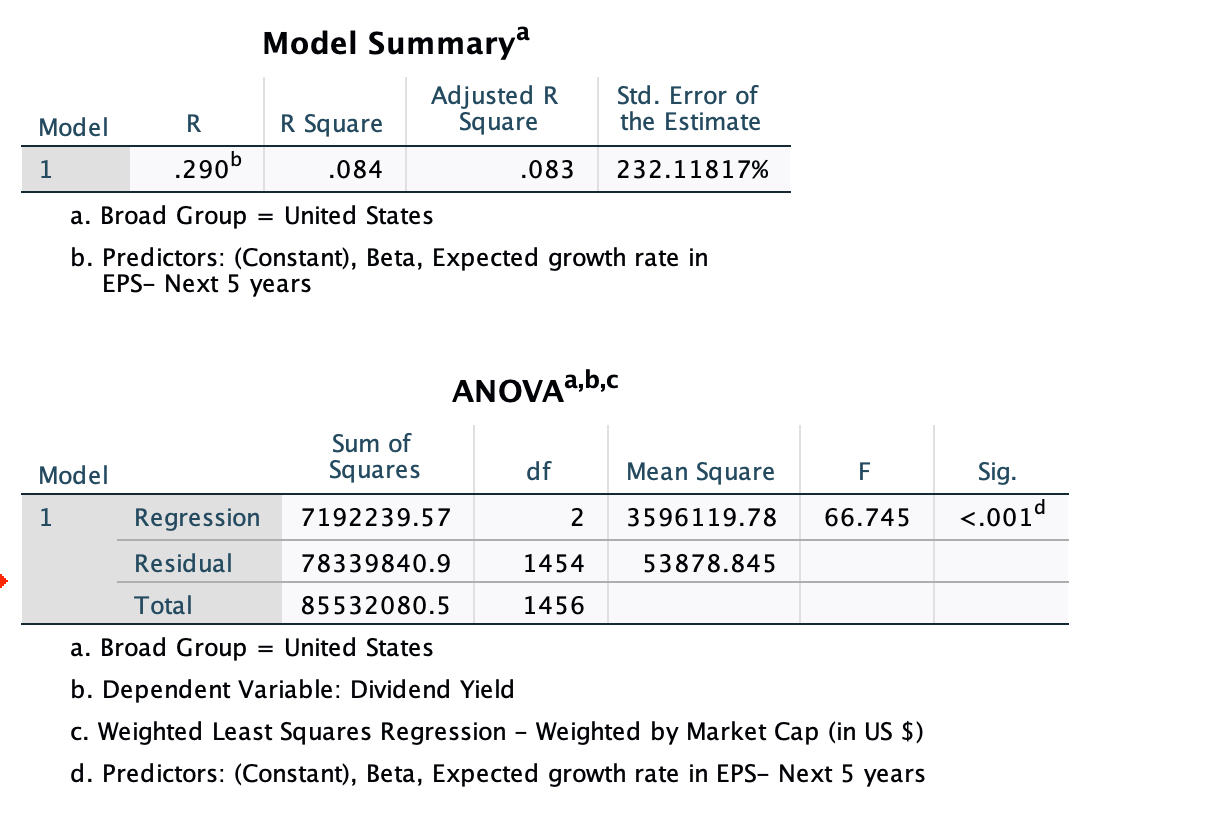### Regression Output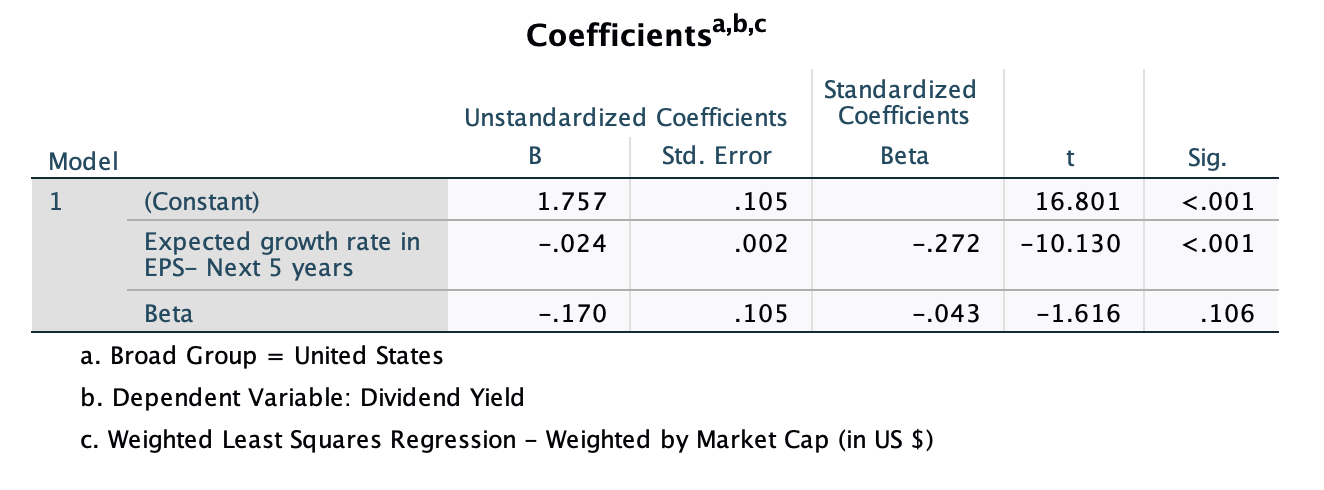US Regression: Dividend Payout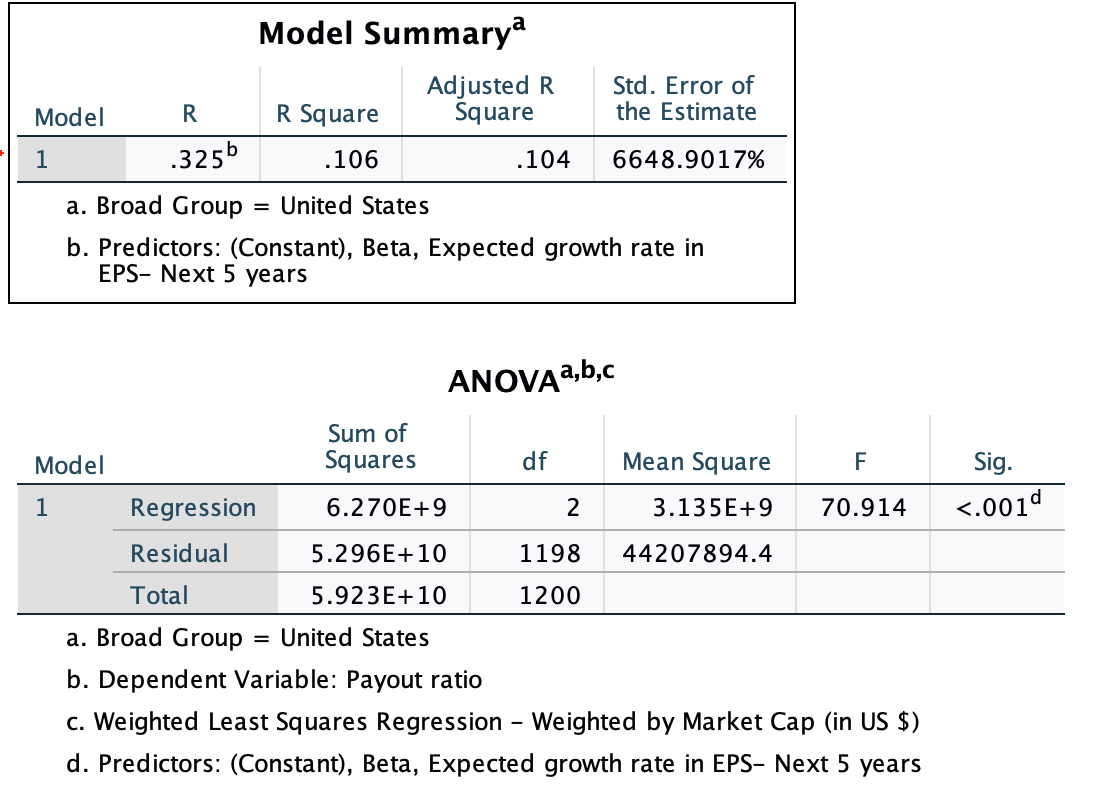### Regression Output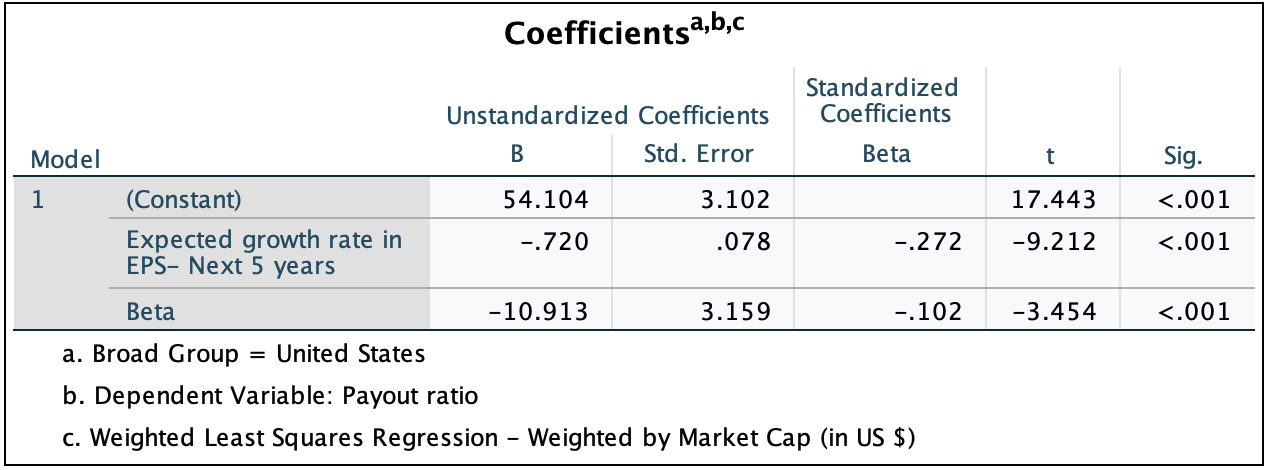Global Regression: Dividend Yield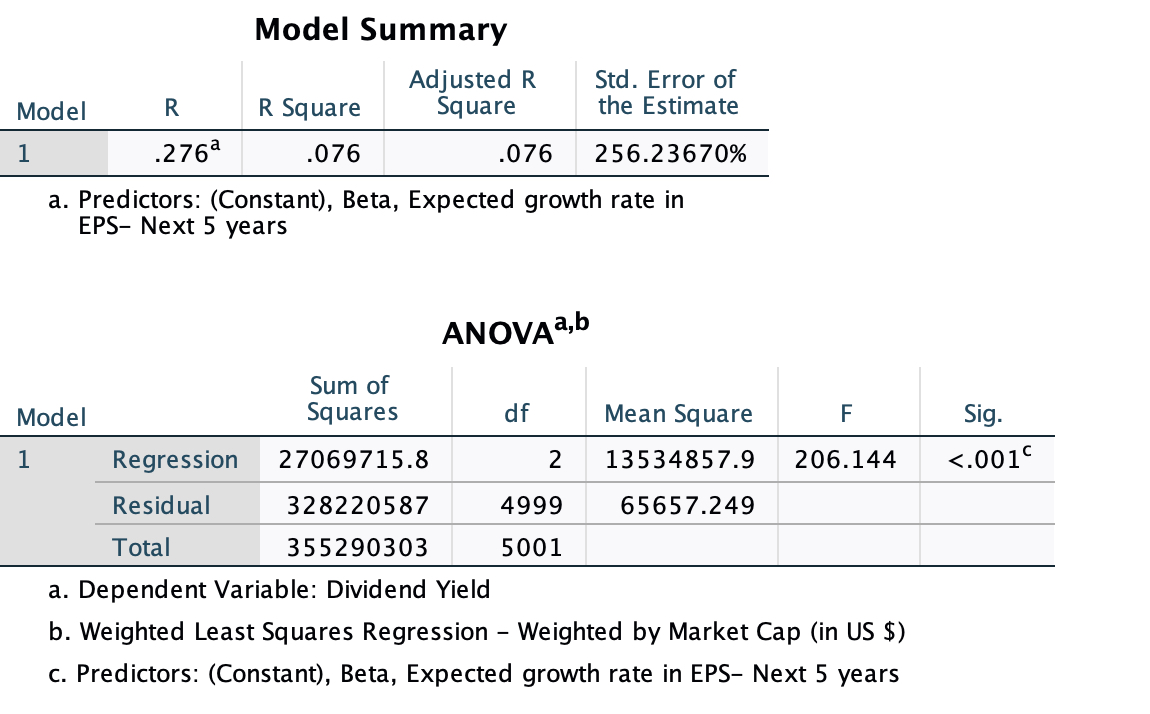### Regression Output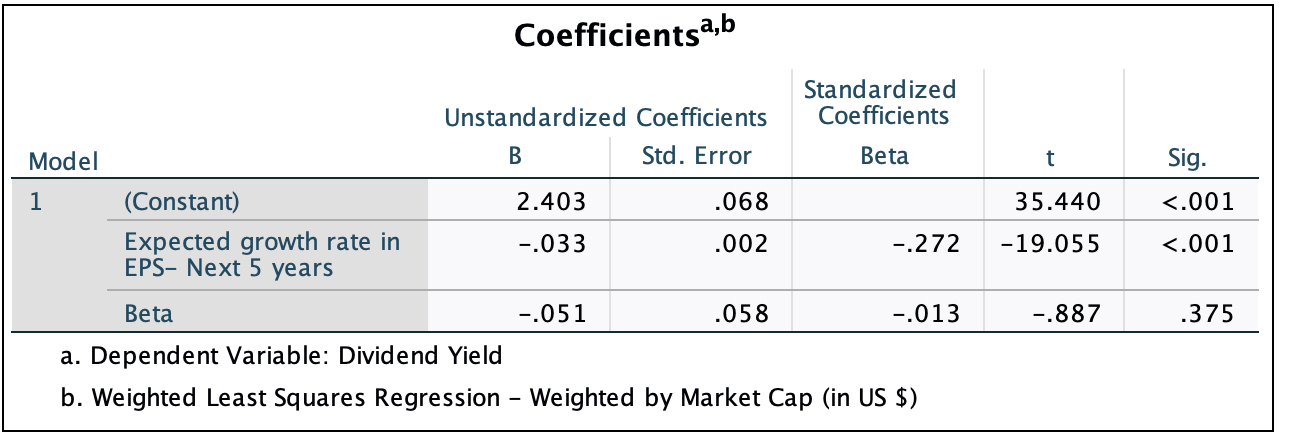Global Regression: Dividend Payout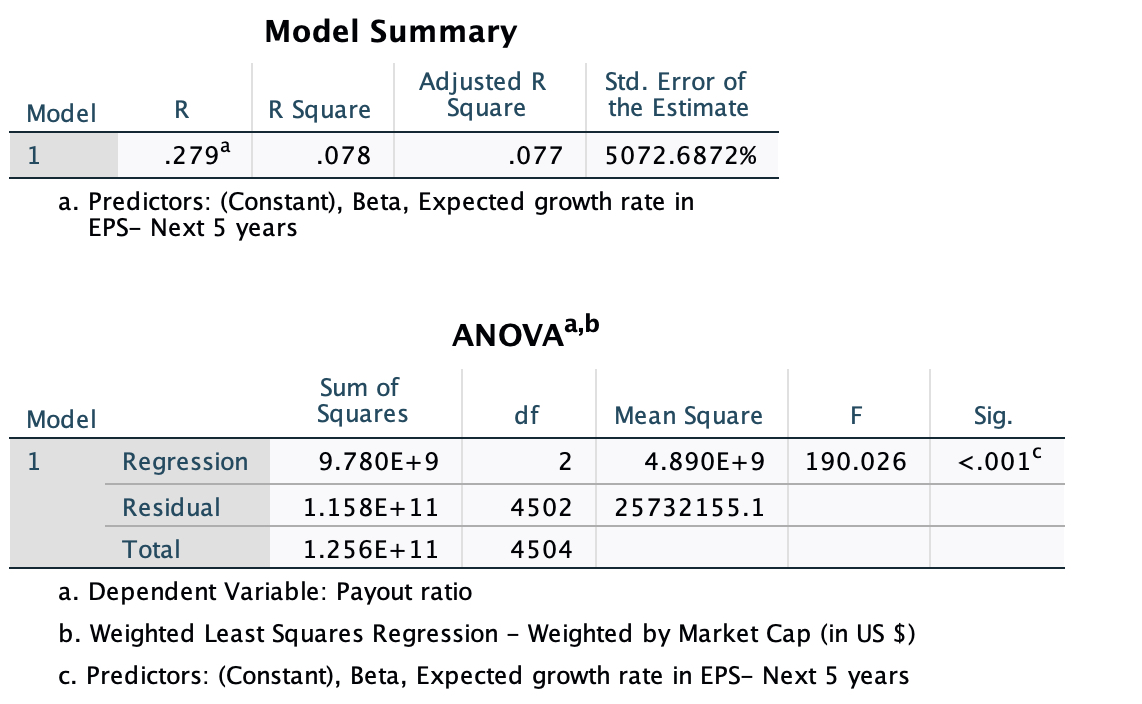### Regression Output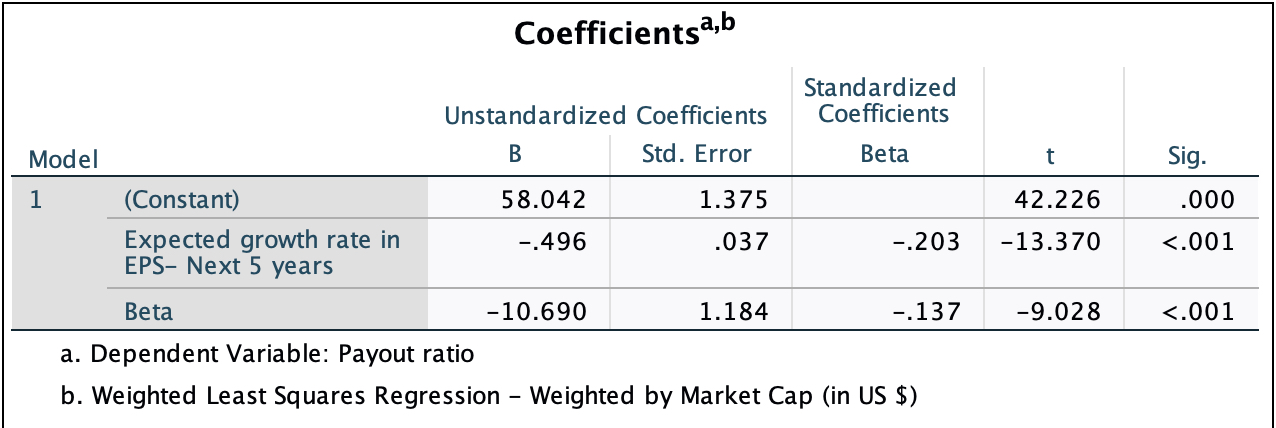• How do I use this regression?

Assume that you want to estimate the dividend payout ratio for a firm with the following characteristics, using the US regression:

Regression beta = 1.20

Expected Growth in EPS over next 5 years = 10%

• Predicted Values
• Expected Dividend Yield =1.76 -0.024 (10) - .17 (1.20) = 1.32 or 1.32%
• Expected Dividend payout ratio = 54.10 - 0.72 (10) - 10.91 (1.20)= 33.80 or 33.80%

If your predicted value is less than zero, your predicted dividend payout ratio is zero.